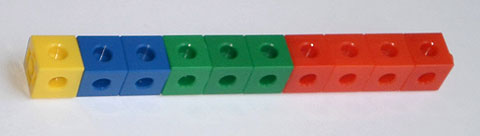Home > Patterns > Big ideas > Growing patterns

# Growing patterns

The row of cubes is made from four chunks, each in a different colour. The lengths of these chunks are 1, 2, 3 and 4 cubes. We expect the next chunk to have 5 cubes, and so on. This is a regular pattern because the chunks get bigger by 1 each time.A growing pattern of cubes.

The row of cubes is an example of a growing pattern. In growing patterns, the chunks are called terms. There has to be a rule that tells you how each term is obtained.

Here is another, more complicated example. The standard paper size, A4, is only one in the international standard A-series of paper sizes.

 Size Dimensions (mm) A0 841 $$\times$$ 1189 A1 594 $$\times$$ 841 A2 420 $$\times$$ 594 A3 297 $$\times$$ 420 A4 210 $$\times$$ 297 A5 148 $$\times$$ 210

These sizes form a growing pattern. (Although the sizes are getting smaller, it is still considered to be a growing pattern.)

Each term is a particular size of paper.

For each term, the length of each side is always $$\sqrt 2$$ (approximately 1.414) times its width.

As a result, each size can be made by folding the previous size in half. Because of this rule, it is possible to continue the series as far as you like.

There is further information about international standard paper and envelope sizes on Wikipedia.

Look at an example of a growing pattern using pattern blocks.

## Making a staircase

Here is an activity to introduce students to the idea of growing patterns.